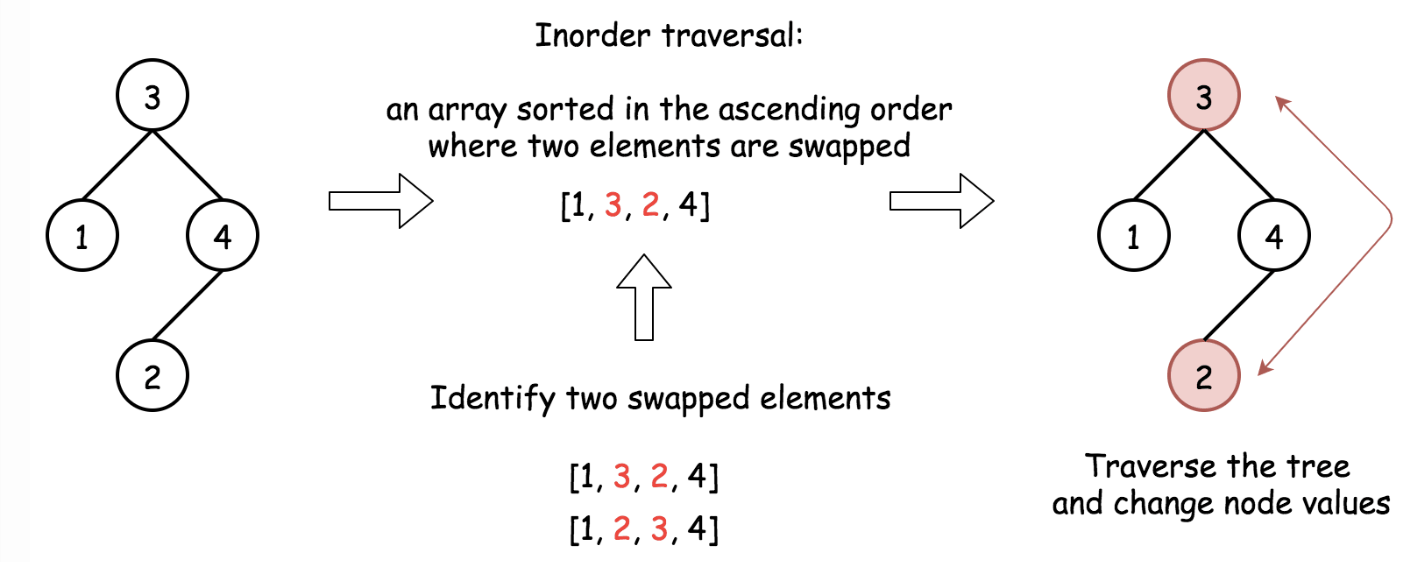# 99. Recover Binary Search Tree

Reference: LeetCode
Difficulty: Hard

## Problem

Two elements of a binary search tree (BST) are swapped by mistake. Recover the tree without changing its structure.

Example:

• A solution using $O(N)$ space is pretty straightforward. Could you devise a constant space solution?

## Analysis

Methods:

1. Sort An Almost Sorted Array
• Construct inorder traversal of the tree. It should be an almost sorted list where only two elements are swapped.
• Identify two swapped elements x and y in an almost sorted array.
• The algorithm assumes that there is only one inversion which allows cases like:
• [3 2 1 4] (it can’t be [3 2 1 0] since it needs more than one swapping)
• [2 1 3 4]
• So, we update y = nums.get(i + 1) first for two times if necessary, and update x = nums.get(i) only once in the first time.
• Traverse the tree again and swap x and y.Time: $O(N)$ since we apply traversals.
Space: $O(N)$
Note: The following approach is based on swapping during inorder traversal. Iterative and recursive approaches here do less than one pass, but they both require up to $O(h)$ space to keep stack. Morris approach needs two passes, but it requires constant space.

1. One-Pass (Iteration)
• To identify swapped nodes, track the last node pred in the inorder traversal and compare it with current node. If the current node is smaller than pred, they are swapped nodes. Then we break the traversal after swapping.

Time: $O(N)$
Space: $O(h)$

1. One-Pass (Recursion)
• Remember to do pred = root!

Time: $O(N)$
Space: $O(h)$

1. Morris Inorder Traversal
• Marked
• Time: $O(N)$
• Space: $O(1)$

CommentJunhao Wang
a software engineering cat